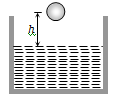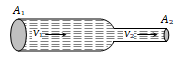A ball of radius r and density $\mathrm{\rho }$ falls freely under gravity through a distance h before entering water. Velocity of ball does not change even on entering water. If viscosity of water is $\mathrm{\eta }$, the value of h is given by(a) $\frac{2}{9}{\mathrm{r}}^{2}\left(\frac{1-\mathrm{\rho }}{\mathrm{\eta }}\right)\mathrm{g}$

(b) $\frac{2}{81}{\mathrm{r}}^{2}\left(\frac{\mathrm{\rho }-1}{\mathrm{\eta }}\right)\mathrm{g}$

(c) $\frac{2}{81}{\mathrm{r}}^{4}{\left(\frac{\mathrm{\rho }-1}{\mathrm{\eta }}\right)}^{2}\mathrm{g}$

(d) $\frac{2}{9}{\mathrm{r}}^{4}{\left(\frac{\mathrm{\rho }-1}{\mathrm{\eta }}\right)}^{2}\mathrm{g}$

Concept Questions :-

Viscosity
High Yielding Test Series + Question Bank - NEET 2020

Difficulty Level:

The rate of steady volume flow of water through a capillary tube of length 'l' and radius 'r' under a pressure difference of P is V. This tube is connected with another tube of the same length but half the radius in series. Then the rate of steady volume flow through them is (The pressure difference across the combination is P)

(a) $\frac{\mathrm{V}}{16}$                                     (b) $\frac{\mathrm{V}}{17}$

(c) $\frac{16\mathrm{V}}{17}$                                    (d) $\frac{17\mathrm{V}}{16}$

Concept Questions :-

Viscosity
High Yielding Test Series + Question Bank - NEET 2020

Difficulty Level:

A liquid is flowing in a horizontal uniform capillary tube under a constant pressure difference P. The value of pressure for which the rate of flow of the liquid is doubled when the radius and length both are doubled is

(a) P                             (b) $\frac{3\mathrm{P}}{4}$

(c) $\frac{\mathrm{P}}{2}$                           (d) $\frac{\mathrm{P}}{4}$

Concept Questions :-

Viscosity
High Yielding Test Series + Question Bank - NEET 2020

Difficulty Level:

We have two (narrow) capillary tubes T1 and T2. Their lengths are l1 and l2 and radii of cross-section are r1 and r2 respectively. The rate of flow of water under a pressure difference P through tube T1 is 8cm3/sec. If l1 = 2l2 and r1 =r2, what will be the rate of flow when the two tubes are connected in series and pressure difference across the combination is same as before (= P)

(a) 4 cm3/sec                           (b) (16/3) cm3/sec

(c) (8/17) cm3/sec                    (d) None of these

Concept Questions :-

Viscosity
High Yielding Test Series + Question Bank - NEET 2020

Difficulty Level:

The Reynolds number of a flow is the ratio of

(a) Gravity to viscous force

(b) Gravity force to pressure force

(c) Inertia forces to viscous force

(d) Viscous forces to pressure forces

Concept Questions :-

Types of flow

Difficulty Level:

Water is flowing through a tube of non-uniform cross-section ratio of the radius at entry and exit end of the pipe is 3 : 2. Then the ratio of velocities at entry and exit of liquid is -

(a) 4 : 9                          (b) 9 : 4
(c) 8 : 27                         (d) 1 : 1

Concept Questions :-

Equation of continuity
High Yielding Test Series + Question Bank - NEET 2020

Difficulty Level:

A liquid flows in a tube from left to right as shown in figure. ${\mathrm{A}}_{1}$ and ${\mathrm{A}}_{2}$ are the cross-sections of the portions of the tube as shown. Then the ratio of speeds ${\mathrm{v}}_{1}/{\mathrm{v}}_{2}$ will be(a) ${\mathrm{A}}_{1}/{\mathrm{A}}_{2}$

(b) ${\mathrm{A}}_{2}/{\mathrm{A}}_{1}$

(c) $\sqrt{{\mathrm{A}}_{2}}/\sqrt{{\mathrm{A}}_{1}}$

(d) $\sqrt{{\mathrm{A}}_{1}}/\sqrt{{\mathrm{A}}_{2}}$

Concept Questions :-

Equation of continuity

Difficulty Level:

An application of Bernoulli's equation for fluid flow is found in

(a) Dynamic lift of an aeroplane

(b) Viscosity meter

(c) Capillary rise

(d) Hydraulic press

Concept Questions :-

Bernoulli theorem

Difficulty Level:

The Working of an atomizer depends upon

(a) Bernoulli's theorem                 (b) Boyle's law
(c) Archimedes principle               (d) Newton's law of motion

Concept Questions :-

Bernoulli theorem

Difficulty Level:

The pans of a physical balance are in equilibrium. Air is blown under the right hand pan; then the right hand pan will

(a) Move up

(b) Move down

(c) Move erratically

(d) Remain at the same level

Concept Questions :-

Bernoulli theorem